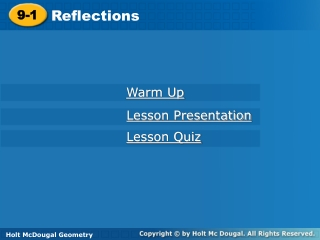DownloadDownload Presentation9-1

9-1

Download Presentation9-1

- - - - - - - - - - - - - - - - - - - - - - - - - - - E N D - - - - - - - - - - - - - - - - - - - - - - - - - - -
Presentation Transcript

1. Reflections 9-1 Warm Up Lesson Presentation Lesson Quiz Holt McDougal Geometry Holt Geometry

2. Warm Up Given that ∆ABC  ∆DEF, identify a segment or angle congruent to each of the following. 1. 2. 3. 4. 5. 6.

3. Objective Identify and draw reflections.

4. Vocabulary isometry

5. An isometry is a transformation that does not change the shape or size of a figure. Reflections, translations, and rotations are all isometries. Isometries are also called congruence transformations or rigid motions. Recall that a reflection is a transformation that moves a figure (the preimage) by flipping it across a line. The reflected figure is called the image. A reflection is an isometry, so the image is always congruent to the preimage.

6. Example 1: Identifying Reflections Tell whether each transformation appears to be a reflection. Explain. B. A. No; the image does not Appear to be flipped. Yes; the image appears to be flipped across a line..

7. Remember! To review basic transformations, see Lesson 1-7, pages 50–55.

8. Check It Out! Example 1 Tell whether each transformation appears to be a reflection. a. b. Yes; the image appears to be flipped across a line. No; the figure does not appear to be flipped.

9. Draw a segment from each vertex of the preimage to the corresponding vertex of the image. Your construction should show that the line of reflection is the perpendicular bisector of every segment connecting a point and its image.

10. Example 2: Drawing Reflections Copy the triangle and the line of reflection. Draw the reflection of the triangle across the line. Step 1 Through each vertex draw a line perpendicular to the line of reflection.

11. Example 2 Continued Step 2 Measure the distance from each vertex to the line of reflection. Locate the image of each vertex on the opposite side of the line of reflection and the same distance from it.

12. Example 2 Continued Step 3 Connect the images of the vertices.

13. Check It Out! Example 2 Copy the quadrilateral and the line of reflection. Draw the reflection of the quadrilateral across the line.

14. 1 Understand the Problem Example 3: Problem-Solving Application Two buildings located at A and B are to be connected to the same point on the water line. Where should they connect so that the least amount of pipe will be used? The problem asks you to locate point X on the water line so that AX + XB has the least value possible.

15. Make a Plan Let B’ be the reflection of point B across the water line. For any point X on the water line, so AX + XB = AX + XB’. AX + XB’ is least when A, X,and B’are collinear. 2 Example 3 Continued

16. 3 Solve Reflect B across the water line to locate B’. Draw and locate X at the intersection of and the water line. Example 3 Continued

17. 4 Look Back Example 3 Continued To verify your answer, choose several possible locations for X and measure the total length of pipe for each location.

18. and would be congruent. Check It Out! Example 3 What if…? If A and B were the same distance from the river, what would be true about and ? A B River X

19. X(2,–1) X’(2, 1) Y(–4,–3) Y’(–4, 3) Z(3, 2) Z’(3, –2) Example 4A: Drawing Reflections in the Coordinate Plane Reflect the figure with the given vertices across the given line. X(2, –1), Y(–4, –3), Z(3, 2); x-axis The reflection of (x, y) is (x,–y). Y’ Z X’ X Z’ Y Graph the image and preimage.

20. S’ T’ R S R(–2, 2) R’(2, –2) T R’ S(5, 0) S’(0, 5) T(3, –1) T’(–1, 3) Example 4B: Drawing Reflections in the Coordinate Plane Reflect the figure with the given vertices across the given line. R(–2, 2), S(5, 0), T(3, –1); y = x The reflection of (x, y) is (y, x). Graph the image and preimage.

21. S V U T S(3, 4) S’(3, –4) T’ U’ T(3, 1) T’(3, –1) U(–2, 1) U’(–2, –1) S’ V’ V(–2, 4) V’(–2, –4) Check It Out! Example 4 Reflect the rectangle with vertices S(3, 4), T(3, 1), U(–2, 1) and V(–2, 4) across the x-axis. The reflection of (x, y) is (x,–y). Graph the image and preimage.

22. Lesson Quiz: Part I 1. Tell whether the transformation appears to be a reflection. yes 2. Copy the figure and the line of reflection. Draw the reflection of the figure across the line.

23. Lesson Quiz: Part II Reflect the figure with the given vertices across the given line. 3.A(2, 3), B(–1, 5), C(4,–1); y = x A’(3, 2), B’(5,–1), C’(–1, 4) 4.U(–8, 2), V(–3, –1), W(3, 3); y-axis U’(8, 2), V’(3, –1), W’(–3, 3) 5.E(–3, –2), F(6, –4), G(–2, 1); x-axis E’(–3, 2), F’(6, 4), G’(–2, –1)